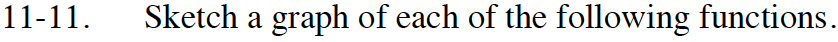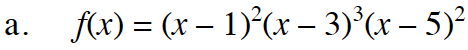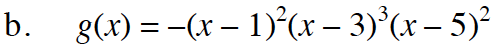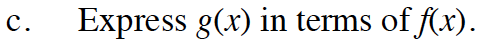Home > INT3 > Chapter 11 > Lesson 11.1.1 > Problem11-11

11-11.
1. Sketch a graph of each of the following functions. Homework Help ✎

1. f(x) = (x – 1)2(x – 3)3(x – 5)2

2. g(x) = –(x – 1)2(x – 3)3(x – 5)2

3. Express g(x) in terms of f(x).There are double roots at 3 points.g(x) = − f(x)